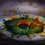# Cauchy Schwarz is too useful...

The famous Cauchy Schwarz Inequality is a very very useful result in $\color{#D61F06}{\textbf{inequalities}}$

Simplest form of it is

$\displaystyle \left| ac+bd \right| \leq \sqrt{a^2+b^2} \sqrt{c^2+d^2}$,

and it's generalized form is

For any two sets of real numbers {$a_1,a_2,a_3,...,a_n$} and {$b_1,b_2,b_3,...,b_n$} , the following inequality holds :-

$\displaystyle \left| \sum_{j=1}^n a_jb_j \right| \leq \biggl( \sum_{j=1}^n a_j^2 \biggr)^{\frac{1}{2}} \biggl( \sum_{j=1}^n b_j^2 \biggr) ^{\frac{1}{2}}$

$\color{#3D99F6}{\textbf{What's important is that}}$

in solving problems, you have to chose your sets {$a_i$} and {$b_i$} $\color{#D61F06}{\textbf{S}} \color{#20A900}{\textbf{M}} \color{#3D99F6}{\textbf{A}}\color{#EC7300}{\textbf{R}}\color{#69047E}{\textbf{T}} \color{limegreen}{\textbf{L}}\color{#95D3FE}{\textbf{Y}}$ to reach your required answer...

For practice, here is one problem (Not made by me, but i changed the numericals than what I had seen). Try this, and it uses the result told above....

For $a,b,c,d \in \mathbb{R} ^+$, prove the following -

$\displaystyle \dfrac{1}{a} +\dfrac{9}{b}+\dfrac{25}{c}+\dfrac{49}{d} \geq \dfrac{256}{a+b+c+d}$Note by Aditya Raut
6 years, 8 months ago

This discussion board is a place to discuss our Daily Challenges and the math and science related to those challenges. Explanations are more than just a solution — they should explain the steps and thinking strategies that you used to obtain the solution. Comments should further the discussion of math and science.

When posting on Brilliant:

• Use the emojis to react to an explanation, whether you're congratulating a job well done , or just really confused .
• Ask specific questions about the challenge or the steps in somebody's explanation. Well-posed questions can add a lot to the discussion, but posting "I don't understand!" doesn't help anyone.
• Try to contribute something new to the discussion, whether it is an extension, generalization or other idea related to the challenge.
• Stay on topic — we're all here to learn more about math and science, not to hear about your favorite get-rich-quick scheme or current world events.

MarkdownAppears as
*italics* or _italics_ italics
**bold** or __bold__ bold
- bulleted- list
• bulleted
• list
1. numbered2. list
1. numbered
2. list
Note: you must add a full line of space before and after lists for them to show up correctly
paragraph 1paragraph 2

paragraph 1

paragraph 2

[example link](https://brilliant.org)example link
> This is a quote
This is a quote
    # I indented these lines
# 4 spaces, and now they show
# up as a code block.

print "hello world"
# I indented these lines
# 4 spaces, and now they show
# up as a code block.

print "hello world"
MathAppears as
Remember to wrap math in $$ ... $$ or $ ... $ to ensure proper formatting.
2 \times 3 $2 \times 3$
2^{34} $2^{34}$
a_{i-1} $a_{i-1}$
\frac{2}{3} $\frac{2}{3}$
\sqrt{2} $\sqrt{2}$
\sum_{i=1}^3 $\sum_{i=1}^3$
\sin \theta $\sin \theta$
\boxed{123} $\boxed{123}$

## Comments

Sort by:

Top Newest

in general, we can just try to prove the Engel form of CS inequality. this will be proved automatically then.

- 6 years, 8 months ago

Log in to reply

$\displaystyle (\sqrt{a}^{2}+\sqrt{b}^{2}+\sqrt{c}^{2}+\sqrt{d}^{2})((\frac{1}{\sqrt{a}})^{2}+(\frac{3}{\sqrt{b}})^{2}+(\frac{5}{\sqrt{c}})^{2}+(\frac{7}{\sqrt{d}})^{2}) \geq (\sqrt{a}\frac{1}{\sqrt{a}}+\sqrt{b}\frac{3}{\sqrt{b}}+\sqrt{c}\frac{5}{\sqrt{c}}+\sqrt{d}\frac{7}{\sqrt{d}})^{2}$

- 6 years, 8 months ago

Log in to reply

lol it went through the space

Do you have any more problems for us to practice Cauchy Schwarz's inequality? Thank you ^__^

- 6 years, 8 months ago

Log in to reply

Yes my friend, I will post some problems rather than notes now :DImgur

- 6 years, 8 months ago

Log in to reply

Thank you meow!!! ^__^

- 6 years, 8 months ago

Log in to reply

×

Problem Loading...

Note Loading...

Set Loading...